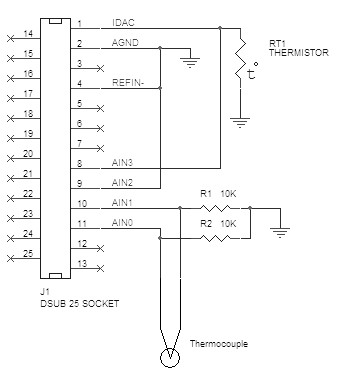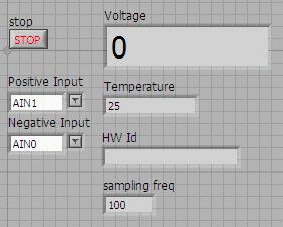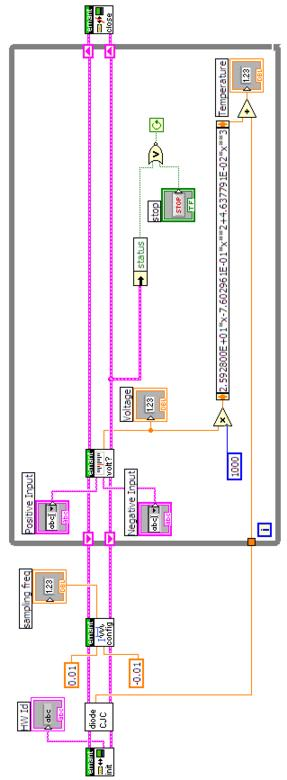# Measure Temperature using Thermocouple

Thermocouples produce an output voltage which depends on the temperature difference between the junctions of two dissimilar metal wires. It is important to appreciate that thermocouples measure the temperature difference between two points, not absolute temperature.

In our example, we measure one of the junctions the cold junction temperature using a thermistor, whilst the other end is attached to a probe. We will use the Type T Thermocouple. The positive conductor is made of copper, and the negative conductor is made of constantan.

The NIST ITS-90 Thermocouple Database gives temperature -- electromotive force (emf) reference functions and tables for the letter-designated thermocouple types B, E, J, K, N, R, S and T. These reference functions have been adopted as standards by the American Society for Testing and Materials (ASTM) and the International Electrotechnical Commission (IEC).Table 1 : Common Thermocouples and their characteristics

The relationship between the temperature difference and the output voltage of a thermocouple is nonlinear and is given by a complex polynomial equation (which is fifth to ninth order depending on thermocouple type).

For the T Type thermocouple, the following information can be obtained from the NIST Thermocouple database

```************************************
* This section contains coefficients of approximate inverse
* functions for type T thermocouples for the subranges of
* temperature and voltage listed below. The range of errors of
* the approximate inverse function for each subrange is also given.
* The coefficients are in units of C and mV and are listed in
* the order of constant term up to the highest order.
* The equation is of the form t_90 = d_0 + d_1*E + d_2*E^2 + ...
*     + d_n*E^n,
* where E is in mV and t_90 is in C.
*
*    Temperature        Voltage            Error
*      range              range            range
*      (C)               (mV)             (C)
*    -200. to 0.     -5.603 to 0.000    -0.02 to 0.04
*     .0 to 400.     0.000 to 20.872    -0.03 to 0.03
********************************************************
Inverse coefficients for type T:
Temperature  -200.             0.
Range:        0.           400.
Voltage   -5.603          0.000
Range:     0.000         20.872
0.0000000E+00  0.000000E+00
2.5949192E+01  2.592800E+01
-2.1316967E-01 -7.602961E-01
7.9018692E-01  4.637791E-02
4.2527777E-01 -2.165394E-03
1.3304473E-01  6.048144E-05
2.0241446E-02 -7.293422E-07
1.2668171E-03  0.000000E+00
Error      -0.02          -0.03
Range:      0.04           0.03
```

Since we are interested in measuring in the room temperature range, we will use the coefficients from the second column.

TC = 2.592800E+01*V -7.602961E-01*V^2 + 4.637791E-02 * V^3

where TC is the temperature in C and V is in mV. For this example, we calculate up to the fourth order (you may modify the VI for the higher orders. Note that LabVIEW provides a subVI in its DAQ library that incorporate this information)

To measure temperature using the T type thermocouple, connect up the circuit as shown. For the EMANT380 Bluetooth DAQ, 3.3V power must be supplied.The LabVIEW VI that measures the temperature using the T type thermocouple is called EMANT300 Example Thermocouple.VI

First the program measures the Cold Junction Temperature using the thermistor (see application note on measuring temperature using Thermistor). After that the programmable gain amplifier (PGA) is set to maximum of 128 using the EMANT300 Configure Analog Simple.VI by setting the low limit and high limit. This is necessary as the maximum voltage from the T thermocouple even at 200C is just 20.872 mV.

From the equation above, the measured voltage must first be converted to mV and then an expression node is used to implement the equation to convert the voltage to temperature.

## Front Panel## Block Diagram